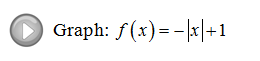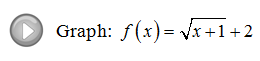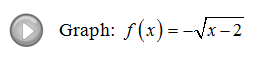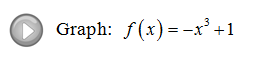## Algebra

Showing posts with label sketch. Show all posts
Showing posts with label sketch. Show all posts

### Hyperbolas

An hyperbola is the set of all points whose distances to two fixed points subtract to the same constant. In this section, we will focus on the equation of hyperbolas.The axis that contains the vertices is called the transverse axis and the axis that does not contain the vertices is called the conjugate axis. Also notice that the shape is very different than that of a parabola, hyperbolas are asymptotic to the following lines:We will make use of these asymptotes to sketch the graphs of hyperbolas.[ Interactive: Hyperbolas ]
To easily graph the asymptotes of a hyperbola use the following process.

Step 1: Identify the center (h, k) from standard form.
Step 2: Plot points a units left and right from center.
Step 3: Plot points b units up and down from center.
Step 4: Draw the rectangle defined by these points.
Step 5: The lines though the corners of this rectangle are the asymptotes!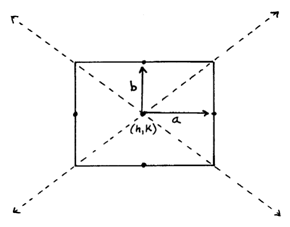Finally use the equation to determine if the parabola is vertical or horizontal.We knew to draw the above hyperbola opening to the left and right because the x^2 term was positive.  If the x^2 term is negative then the hyperbola opens up and down.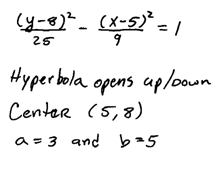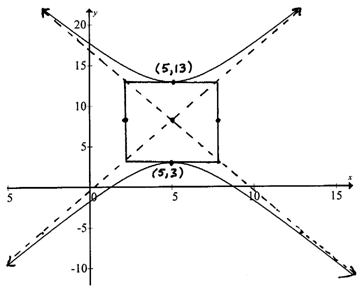Compare this hyperbola to the previous and note the difference in standard form. The rectangle and asymptotes are not actually part of the graph. We use these to obtain a more accurate sketch.  When graphed on a graphing utility the result looks like this.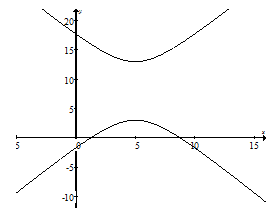Graph the hyperbola and give the equations of the asymptotes.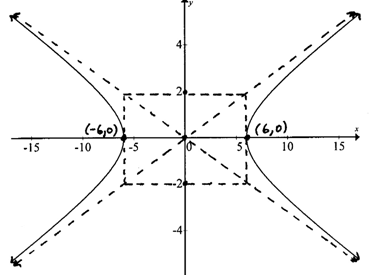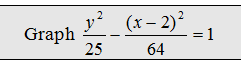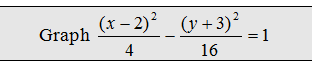The next examples require us to complete the square to obtain standard form.  Remember to factor the leading coefficient out of each variable grouping before using (B/2)^2 to complete the square.

Graph the hyperbola, x- and y- intercepts, and give the equations of the asymptotes.When we completed the square, notice that we added 64 and subtracted 225 to balance the equation.  It may appear, at first glance, that we added 16 and 25 to the left side. But in reality, after we distribute the 4 and -9 we added and subtracted larger values.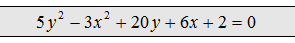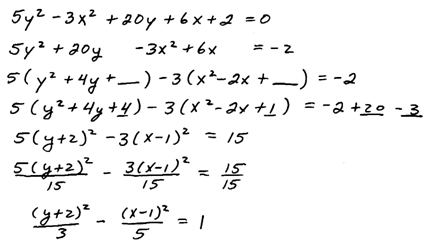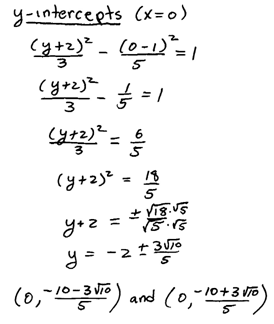When the transverse axis is vertical be careful with the center.  A common error is to use (-2, 1) for the center in the above example because the y variable comes first and we are used to reading these from left to right.  This would be incorrect so take care to use the correct h and k.

Example: Find the equation of the hyperbola centered at (-3, 1) where a = 2, b = 3, and with a vertical transverse axis.  Graph it.Example: Find the equation of the hyperbola with vertices (3,0) and (-3,0), horizontal transverse axis, and conjugate axis of length 4 units.Example: Find the equation of the hyperbola with center (2, -5), vertical transverse axis measuring 10 units, and conjugate axis measuring 6 units.YouTube Videos: Click problem to see it worked out.

---

### Graphing Functions using Transformations

One way to graph functions is to simply plot points.  In this section, we cover a method used to quickly sketch graphs related to some basic functions. Here we focus on rigid transformations, that is, transformations that do not change the shape of the graph.

Vertical Translations: [ Interactive Graph ]
If k is any positive real number then,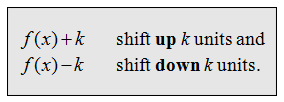The graph of the basic function f(x) = sqrt(x) follows:Using this basic graph and the vertical translations described above we can sketch f(x) = sqrt(x) + 2 by shifting all of the points up 2 units. Similarly, graph g(x) = sqrt(x 3 by shifting all points down 3 units.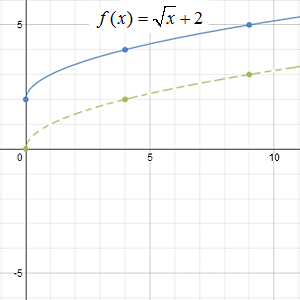Horizontal Translations:  [ Interactive Graph ]
If h is any positive real number then,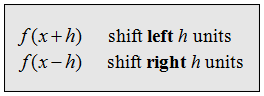Using the graph of f(x) = sqrt(x) and the horizontal translations described above we can sketch f(x) = sqrt(x + 4) by shifting all of the points left 4 units. Similarly, graph g(x) = sqrt( 3) by shifting all points right 3 units.Reflections: [ Interactive Graph ]
Given any function f(x),Using the graph of f(x) = sqrt(x) sketch the graph of f(x) = −sqrt(x)  by reflecting all of the points about the x-axis. Similarly, graph f(x) = sqrt(−x) by reflecting all of the points about the y-axis.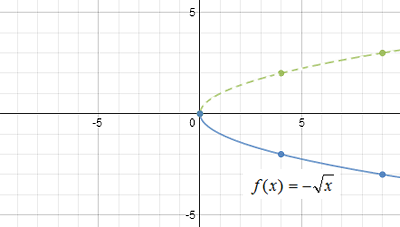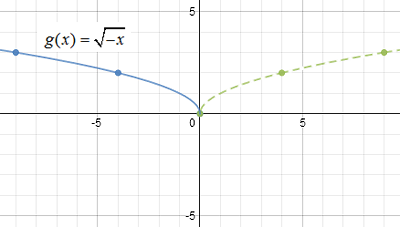For the first function f(x) = −sqrt(x) all of the y-values are negative which results in a reflection about the x-axis. For the second function f(x) = sqrt(−x) all of the x-values must be negative thus resulting in a reflection about the y-axis.
Sketch the graph.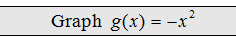The -1 indicates a reflection of the graph of the squaring function  f(x) = x^2 about the x-axis. Be sure to graph the squaring function using a dashed curve because it will be used as a guide and is not the answer. Next, reflect all points about the x-axis and draw in the final graph with a solid curve.General Steps for Graphing Functions using Transformations:
1. Identify and graph the basic function using a dashed curve.
2. Identify any reflections first and sketch them using the basic function as a guide.
3. Identify any translations.
4. Use this information to sketch the final graph using a solid curve.

Graph the function and determine the domain and range.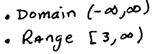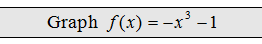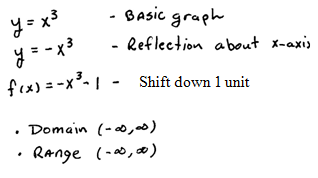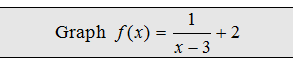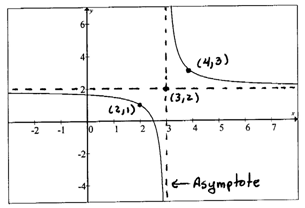Use the transformations to determine the equation that represents the given function.

Example: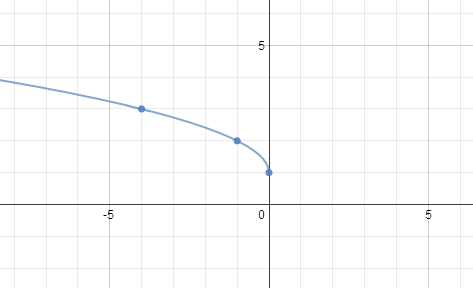From the general shape of the graph we can determine the basic function and transformations. The graph has the shape of the square root function,
y = sqrt(x)
Next, notice the reflection about the y-axis,
y = sqrt(x)
And finally, we see a shift up 1 unit.
y = sqrt(x) + 1Example:The given function has the general shape of the squaring function (parabola),
y = x^2
Next, notice the shift right 3 units,
y = ( 3)^
And finally, we see a shift down 2 units.
y = ( 3)^ 2Example:The given function has the general shape of the absolute value function,
y = abs(x)
Next, notice the reflection about the x-axis,
y = abs(x
Finally we see a shift left 1 unit and down 2 units
y = abs(x+1) − 2You Tube Videos: